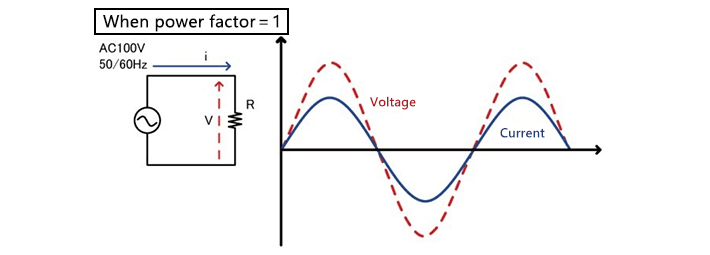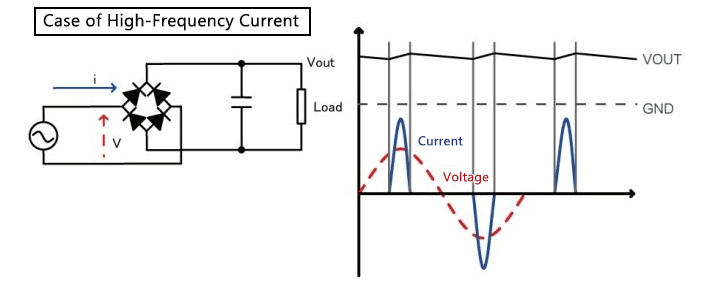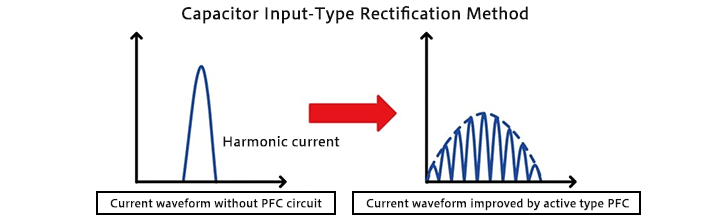Technical Information Site of Power Supply Design

• TECH INFO
• Is Power Supply Efficiency Always Reduced When a PFC Circuit is Added?

AC/DC Converter Control that Achieves Both Power Factor Correction and High Efficiency

# Is Power Supply Efficiency Always Reduced When a PFC Circuit is Added?

Keyword EfficiencyPower factorOutput power as a fraction of input powerEffective power as a fraction of apparent powerSwitching power supplyHigh-frequency componentRestricting high-frequency currentPower factor correction (PFC) circuitPassive type PFCActive type PFCSine wavePFC controllerBM1C101FQuasi-resonant designEnergy Star 6.0

Energy conservation is today an urgent issue throughout the world, and there is a demand for electronic equipment with reduced power consumption not only during operation, but while in standby mode as well. Moreover, in order to reduce waste of supplied power, PFC (power factor correction) functions are indispensable. However, because efficiency is reduced due to PFC, particularly during standby and light loading, device designs that simultaneously achieve a high power factor and high efficiency are a major challenge.

Given this situation, ROHM developed AC/DC converter ICs that utilize unique power supply control technology to simultaneously achieve device power factor correction and high efficiency during standby. We asked a ROHM engineer involved in the development about the problems faced in power factor improvement and higher efficiency, and the company's approach to their resolution.

－The theme for today is the "simultaneous achievement of power factor correction and high efficiency under light loading". I'd like to begin by asking you to explain "power factor" and "efficiency" in simple terms.

In recent years the term "efficiency" has been used frequently, but ordinarily people probably never use the term "power factor".

Efficiency is the output power as a fraction of the input power, nearly always expressed in percent. As a simple example, if the output power of a power supply is 60 W as a result of an input power (power consumption) of 80 W, then the ratio 60 W/80 W means an efficiency of 75%. This is easy to understand.

Power factor may be a bit more troublesome, since it requires an understanding of AC apparent power, effective power, and reactive power. The power factor itself is the effective power as a fraction of the apparent power, at maximum equal to 1. Values of the power factor are typically expressed as decimals such as 0.9 or 0.95. The apparent power is the power transmitted by a power company, and is what "appears to be" the power. The effective power is the power used by devices, that is, by a load. For example, referring again to the earlier example of the power supply, if the power (effective power) that is used (consumed) by the power supply (device) is 80 W, and if the power transmitted by the power company (the apparent power) to supply this 80 W is 100 W, then the power factor is 80 W/100 W, or 0.8.

The power factor is a quantity of AC power, and so is considered in terms of vectors. If the phase difference between the voltage and current in AC power is θ, then the power factor can be computed as cos θ; if the voltage and current are sine waves and there is no phase difference between them, then the power factor is 1. A text explanation is harder to follow, so let's look at some graphs.When the load is a simple resistance, a phase lag does not occur, and so the power factor is 1. In the case of a capacitive or an inductive load, or when the waveform is not sinusoidal, a phase shift occurs, and the power factor is no longer 1. Let us consider this in terms of the input voltage and current waveform of a switching AC/DC converter, which is the theme of this article.

In a switching power supply, a capacitor is used to smooth the input AC voltage (this is called capacitor input-type rectification/smoothing). This smoothing capacitor is, of course, a capacitive load. The alternating current input to this capacitor flows only when the input AC voltage is higher than the capacitor voltage, and therefore becomes a current that contains pulse-like high-frequency components with a small conduction angle that are not sinusoidal, as shown in the diagram below.In such a state, even if the power consumption is the same, a large current flows temporarily on the power supply side. Hence if Cin = 100 μF, Vin = 100 Vrms, and Po = 20 W, then the peak value of the input current is 1.4 A. The power factor is then approximately 0.5, and the input current becomes 5 times larger than the value when the power factor is 1. Hence for a load in which such a current flows, that is non-sinusoidal and includes high-frequency components, a large apparent power becomes necessary. Moreover, the power company that supplies the power must prepare facilities for generation of the extra power and to handle large power peaks, so that costs are increased.

－To put it simply, then, if the power factor of the power supply of a device is poor, more power than is consumed by the device must be applied, resulting in excessive waste in power generation and transmission.

That's right. The problem of poor power factors is already been addressed in countries around the world, and quite a few countries are introducing relevant regulations, such as to restrict high-frequency currents in equipment that consumes a specified amount of power or more. One means of dealing with these regulations is to use power factor correction (PFC) circuits, to raise the power factor and suppress high-frequency currents. In Europe, equipment rated at 75 W and above is required to be equipped with PFC, and in Japan also, PFC circuits are almost always installed.

－How does PFC improve the power factor?

PFC is generally of the passive type, using a passive element (inductor), or of the active type, using power switches such as MOSFETs. The diagram below is a conceptual illustration of improvement of a current waveform by an active type PFC circuit. A pulse-like current waveform having high peaks is chopped by switching operation to approximate a sine wave.Characteristics of a passive-type circuit include a simple configuration, but difficulty in dealing with a broad range of input voltages, and difficulty of miniaturization as well. On the other hand, active-type circuits can handle a broad range of input voltages, and are suited to miniaturization. ICs that are called PFC controllers are, of course, of the active type.

－Well then, I'd like to move on to "simultaneously achieving power factor improvement and high efficiency", which is the theme of this article. Does this mean that "if a PFC circuit is installed, efficiency drops"?

When installing an active PFC circuit, power consumption increases by the amount of the circuit, so efficiency is reduced. In particular, in electronic equipment of recent years that has a standby mode, the effect exerted by the power consumption of the PFC circuit on the standby efficiency is prominent.

The BM1C101F is a controller IC for AC/DC converters, incorporating PFC control functions, that was developed to address these issues. The BM1C101F employs a quasi-resonant design, and features a function for turn on/off of the internal PFC controller at preset power, and a new control design enabling switching of the PFC output. Through these features, power in standby mode can be greatly reduced, and the device satisfies the requirements of the Energy Star 6.0 international standard.

## AC/DC Converter Control that Achieves Both Power Factor Correction and High Efficiency